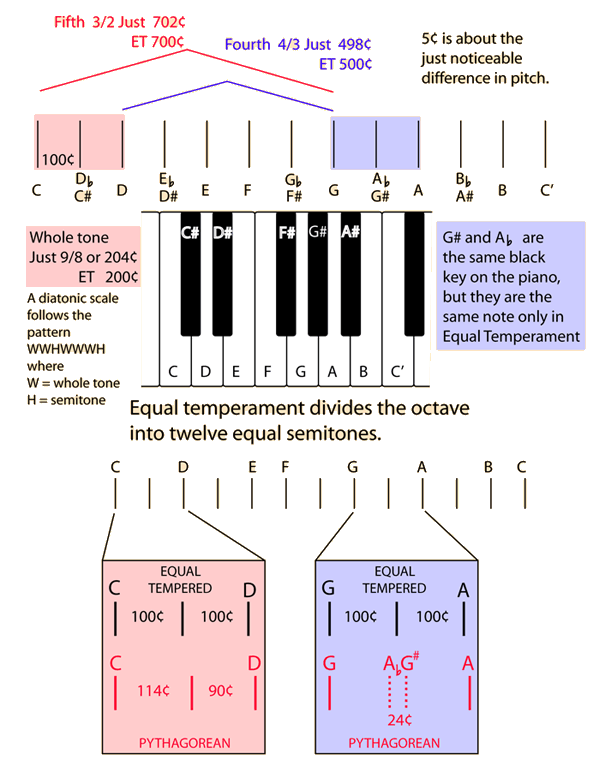# Ideas associated with the equal tempered octave

The equal tempered scale is the common musical scale used at present, used for the tuning of pianos and other instruments of relatively fixed scale. The middle octave of the piano is illustrated below and used to point to some of the features of equal tempered intervals compared to Just and Pythagorean intervals.Equal temperament divides the octave into 12 equal semitones. It is common practice to state musical intervals in cents, where 100˘ is defined as one equal tempered semitone. The cents notation provides a useful way to compare intervals in different temperaments and to decide whether those differences are musically significant. A useful parameter for comparison is the just noticeable difference (JND) in pitch which corresponds to about 5˘.

If the 5˘ JND in pitch is used as the criterion for acceptable tuning accuracy, then it can be seen that Equal Temperament holds the fifth, the fourth and the whole tone to acceptable accuracy. The breaking of the whole tone into semitones differs significantly from Pythagorean temperament as shown above. The equal tempered major and minor thirds differ significantly from the small whole number ratios of just temperament, and significantly affect the tuning of the major triad.

 Equal tempered frequency list
 Equal tempered octave on the piano
Index

Temperament and musical scales

 HyperPhysics***** Sound R Nave
Go Back